# 用python实现3D花灯

dmzhaoq1

0收藏

1 前言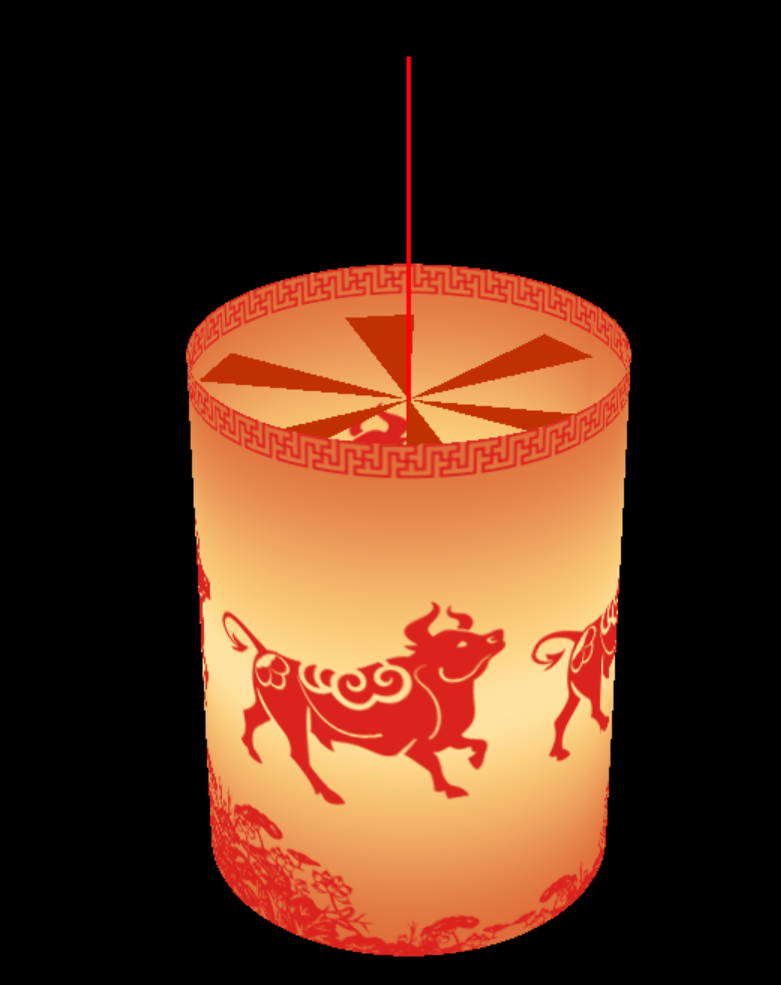2 原材料

2.1 花灯纸2.2 Python环境和模块

numpy
pillow
wxgl

``````pip install numpy
pip install pillow
pip install wxgl
``````

NumPy和pillow是Python旗下最常用的科学计算库和图像处理库，属于常用模块。WxGL是一个基于PyOpenGL的三维数据可视化库，以wx为显示后端，提供Matplotlib风格的交互式应用模式，同时，也可以和wxPython无缝结合，在wx的窗体上绘制三维模型。

3 制作工序

3.1 导入模块

``````>>> import numpy as np
>>> from PIL import Image
>>> import wxgl.wxplot as plt
``````

3.2 打开花灯纸图像

``````>>> fn = r'D:\temp\light0115\res\paper.png'
>>> im = np.array(Image.open(fn))/255
>>> im.shape
(400, 942, 3)
``````

fn定义的是图像存储路径，请据实修改。Image.open(fn)打开文件，返回一个PIL对象，np.array()将PIL对象转成numpy.ndarray数组对象。除以255，将图像数据从0到255的值域范围变成0到1，适应WxGL的接口要求。查看数组的shape，显示图像分辨率为400像素高、942像素宽，每个像素有三种颜色（此处为RGB）。

3.3 根据花灯纸的大小制作龙骨

``````>>> rows, cols, deep = im.shape
>>> cols/(2*np.pi)
149.9239563925654
>>> r = 1
>>> h = 2*np.pi*rows/cols
>>> h
2.6680192387174464
``````

``````>>> theta = np.linspace(0, 2*np.pi, cols)
>>> x = r * np.cos(theta)
>>> y = r * np.sin(theta)
>>> z = np.linspace(0, h, rows)
>>> xs = np.tile(x, (rows,1))
>>> ys = np.tile(y, (rows,1))
>>> zs = z.repeat(cols).reshape((rows,cols))
``````

``````>>> plt.mesh(xs[::10,::10], ys[::10,::10], zs[::10,::10], mode='FLBL')
>>> plt.show()
``````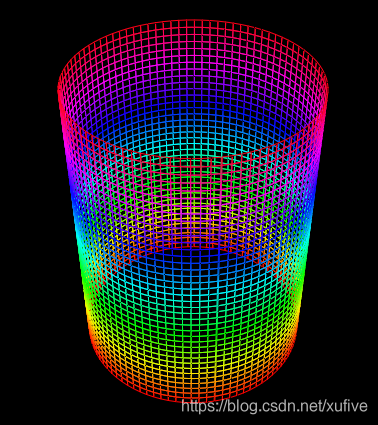3.4 给龙骨贴上花灯纸

``````>>> plt.mesh(xs, ys, zs, im)
>>> plt.show()
``````

``````>>> plt.mesh(xs, ys, zs, im[::-1])
>>> plt.show()
``````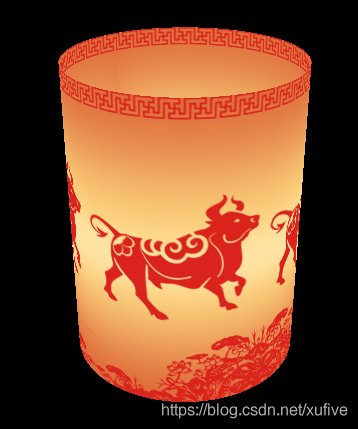3.5 制作旋转叶轮

``````>>> theta = np.linspace(0, 2*np.pi, 18, endpoint=False)
>>> x = r * np.cos(theta)
>>> y = r * np.sin(theta)
>>> x[2::3] = x[1::3]
>>> x[1::3] = 0
>>> y[2::3] = y[1::3]
>>> y[1::3] = 0
>>> z = np.ones(18) * h * 0.9
>>> vs = np.stack((x,y,z), axis=1)
>>> plt.mesh(xs, ys, zs, im[::-1])
>>> plt.surface(vs, color='#C03000', method='T', mode='FCBC', alpha=0.8)
>>> plt.show()
``````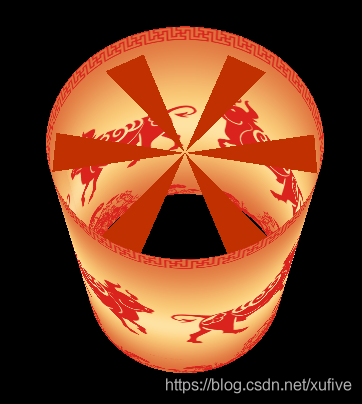3.6 加上照明灯和提系

``````>>> plt.mesh(xs, ys, zs, im[::-1])
>>> plt.surface(vs, color='#C03000', method='T', mode='FCBC', alpha=0.8)
>>> plt.sphere((0,0,h*0.4), 0.4, '#FFFFFF', slices=60, mode='FCBC')
>>> plt.plot((0,0), (0,0), (0.4*h, 1.5*h), width=3.0, style='solid', cmap='hsv', caxis='z')
``````

3.7 让花灯转起来

``````plt.show(rotation='h-')
``````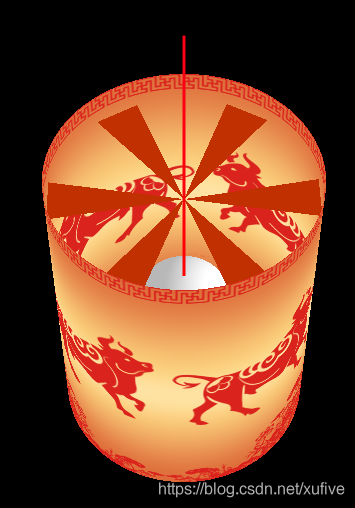4 完整源代码

``````# -*- coding: utf-8 -*-

import numpy as np
from PIL import Image
import wxgl.wxplot as plt

im = np.array(Image.open('res/paper.png'))/255
rows, cols, deep = im.shape

r, h = 1, 2*np.pi*rows/cols
theta = np.linspace(0, 2*np.pi, cols)
x = r*np.cos(theta)
y = r*np.sin(theta)
z = np.linspace(0, h, rows)
xs = np.tile(x, (rows,1))
ys = np.tile(y, (rows,1))
zs = z.repeat(cols).reshape((rows,cols))

theta = np.linspace(0, 2*np.pi, 18, endpoint=False)
x = r*np.cos(theta)
y = r*np.sin(theta)
x[2::3] = x[1::3]
x[1::3] = 0
y[2::3] = y[1::3]
y[1::3] = 0
z = np.ones(18) * h * 0.9
vs = np.stack((x,y,z), axis=1)

plt.mesh(xs, ys, zs, im[::-1])
plt.surface(vs, color='#C03000', method='T', mode='FCBC', alpha=0.8)
plt.sphere((0,0,h*0.4), 0.4, '#FFFFFF', slices=60, mode='FCBC')
plt.plot((0,0), (0,0), (0.4*h, 1.5*h), width=3.0, style='solid', cmap='hsv', caxis='z')
plt.show(rotation='h-')
``````

————————————————帖子
视频
声望
粉丝
最近发布
社区精华内容